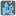• # More tests

## Question related to missionHaunted House

I've created two solutions.

In my initial algorithm, I use a simple breadth first search, and my second solution I calculated all possible states (location Stephan, location ghosts). The later is possible because the number of states is at most 16*16 = 256 (in reality only 128 since the distance between the two always remain either even or odd).

I consider the first solution poor. If the ghost can take two or more moves, this algorithm tries both. Since BFS it is looking for the shortest solution, this means the algorithm favours the shortest route to the exit, which is the option where the ghost makes the most positive for Stephan. While this will often work, a better algorithm would be one where Stephan assumes the worst move by the ghost instead of the best possible move by the ghost.

In practise, the first algorithm succeeds in about 75% of the cases for the second scenario, and fails in about 25%.

```+    +----+----+----+
| G                 |
+    +    +    +    +
|    |    |    |    |
+    +----+----+    +
|    |    |    |    |
+    +    +    +    +
|                S  |
+----+----+----+----+
```

Clearly, my first solution was not good enough, and I like to see a test which will filter it out. Would it be possible to create a scenario where my first solution always fails?